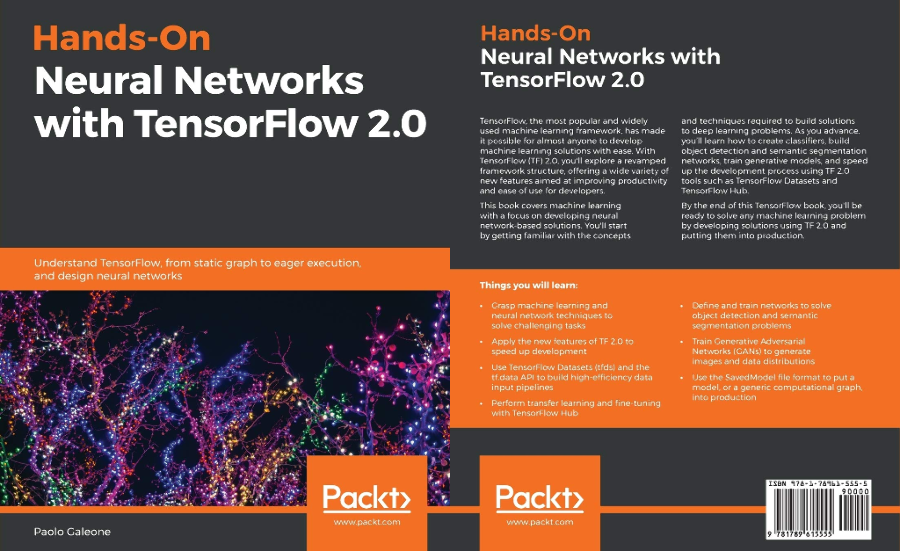# Dissecting tf.function to discover AutoGraph strengths and subtleties

How to correctly write graph-convertible functions in TensorFlow 2.0.Computer engineer | Head of ML & CV @ ZURU Tech Italy | Machine Learning GDE

## TensorFlow 2.0 & DataFlow Graphs

• Execution Speed
• Language Agnostic Representation
• Easy to replicate and distribute
• Automatic Differentiation

## tf.function and AutoGraph

``````# tf.function signature: it is a decorator.
def function(func=None,
input_signature=None,
autograph=True,
experimental_autograph_options=None)
``````

tf.function uses AutoGraph

AutoGraph converts Python control flow statements into appropriate TensorFlow graph ops.

## tf.function and AutoGraph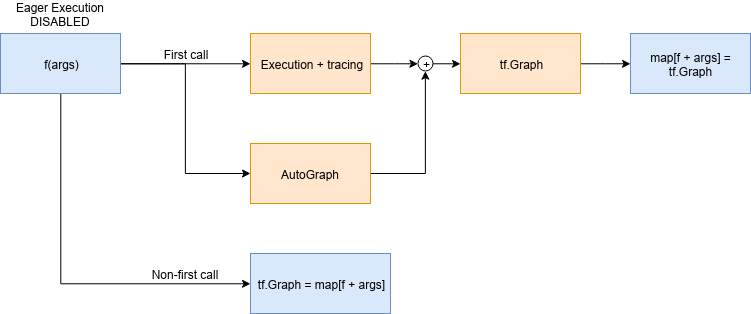## The problem

Given the constant matrices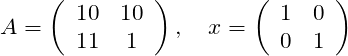And the scalar variable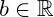Compute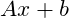## TensorFlow 1.x solution

``````g = tf.Graph()
with g.as_default():
a = tf.constant([[10,10],[11.,1.]])
x = tf.constant([[1.,0.],[0.,1.]])
b = tf.Variable(12.)
y = tf.matmul(a, x) + b
init_op = tf.global_variables_initializer()

with tf.Session() as sess:
sess.run(init_op)
print(sess.run(y))
``````

## TensorFlow 2.0 solution: eager execution

``````def f():
a = tf.constant([[10,10],[11.,1.]])
x = tf.constant([[1.,0.],[0.,1.]])
b = tf.Variable(12.)
y = tf.matmul(a, x) + b
return y
print([f().numpy() for _ in range(10)])
``````

Every tf.* op, produces a `tf.Tensor` object

## From eager function to tf.function

``````@tf.function
def f():
a = tf.constant([[10,10],[11.,1.]])
x = tf.constant([[1.,0.],[0.,1.]])
b = tf.Variable(12.)
y = tf.matmul(a, x) + b
print("PRINT: ", y)
tf.print("TF-PRINT: ", y)
return y

f()
``````

## From eager function to tf.function

``````PRINT:  Tensor("add:0", shape=(2, 2), dtype=float32)
``````

## From eager function to tf.function

``````ValueError: tf.function-decorated function tried to create variables on non-first call.
``````## Lesson #1: functions that create a state

A `tf.Variable` object in eager mode is just a Python object that gets destroyed as soon as it goes out of scope.

​​​

A `tf.Variable` object in a tf.function-decorated function is the definition of a node in a persistent graph (eager execution disabled).

## The solution

``````class F():
def __init__(self):
self._b = None

@tf.function
def __call__(self):
a = tf.constant([[10, 10], [11., 1.]])
x = tf.constant([[1., 0.], [0., 1.]])
if self._b is None:
self._b = tf.Variable(12.)
y = tf.matmul(a, x) + self._b
print("PRINT: ", y)
tf.print("TF-PRINT: ", y)
return y

f = F()
f()
``````

## Lesson #2: eager function is not graph-convertible as is

There is no 1:1 match between eager execution and the graph built by `@tf.function`.

Define the function thinking about the graph that is being built.

# Change the input type

• Python is a dynamically-typed language
• TensorFlow is a strictly statically typed framework

## The function

``````@tf.function
def f(x):
print("Python execution: ", x)
tf.print("Graph execution: ", x)
return x
``````

The function parameters type is used to create a graph, that is a statically typed object, and to assign it an ID

## tf.Tensor as input

``````print("##### float32 test #####")
a = tf.constant(1, dtype=tf.float32)
print("first call")
f(a)
a = tf.constant(1.1, dtype=tf.float32)
print("second call")
f(a)

print("##### uint8 test #####")

b = tf.constant(2, dtype=tf.uint8)
print("first call")
f(b)
b = tf.constant(3, dtype=tf.uint8)
print("second call")
f(b)
``````

## tf.Tensor as input

``````##### float32 test #####
first call
Python execution:  Tensor("x:0", shape=(), dtype=float32)
Graph execution:  1
second call
Graph execution:  1.1

##### uint8 test #####
first call
Python execution:  Tensor("x:0", shape=(), dtype=uint8)
Graph execution:  2
second call
Graph execution:  3
``````

Everything goes as we expect

## Inspecting the function

``````tf.autograph.to_code(f.python_function)
``````
``````def tf__f(x):
try:
with ag__.function_scope('f'):
do_return = False
retval_ = None
with ag__.utils.control_dependency_on_returns(ag__.converted_call(print, None, ag__.ConversionOptions(recursive=True, force_conversion=False, optional_features=ag__.Feature.ALL, internal_convert_user_code=True), ('Python execution: ', x), {})):
tf_1, x_1 = ag__.utils.alias_tensors(tf, x)
with ag__.utils.control_dependency_on_returns(ag__.converted_call('print', tf_1, ag__.ConversionOptions(recursive=True, force_conversion=False, optional_features=ag__.Feature.ALL, internal_convert_user_code=True), ('Graph execution: ', x_1), {})):
x_2 = ag__.utils.alias_tensors(x_1)
do_return = True
retval_ = x_1
return retval_
except:
ag__.rewrite_graph_construction_error(ag_source_map__)
``````

## Inspecting the function

``````with ag__.utils.control_dependency_on_returns(
ag__.converted_call(
print, None, ag__.ConversionOptions(
recursive=True,
force_conversion=False,
optional_features=ag__.Feature.ALL,
internal_convert_user_code=True),
('Python execution: ', x), {})
):
``````

`converted_call` `owner` parameter is `None`: this line becomes a `tf.no_op()`

## Python native type as input

``````def printinfo(x):
print("Type: ", type(x), " value: ", x)

print("##### int test #####")
print("first call")
a = 1
printinfo(a)
f(a)
print("second call")
b = 2
printinfo(b)
f(b)

print("##### float test #####")
print("first call")
a = 1.0
printinfo(a)
f(a)
print("second call")
b = 2.0
printinfo(b)
f(b)
``````

## Python native type as input

### Call with Python int

``````##### int test #####
first call
Type:  <class 'int'>  value:  1
Python execution:  1
Graph execution:  1

second call
Type:  <class 'int'>  value:  2
Python execution:  2
Graph execution:  2
``````

The graph is being recreated at every function invocation

## Python native type as input

### Call with Python float

``````##### float test #####
first call
Type:  <class 'float'>  value:  1.0
Graph execution:  1
second call
Type:  <class 'float'>  value:  2.0
Graph execution:  2
``````
• The return type is WRONG.
• The graphs built for the integers 1 and 2 is reused for the float 1.0 and 2.0## Lesson #3: no autoboxing

`tf.function` does not automatically convert a Python integer to a `tf.Tensor` with dtype `tf.int64`, and so on.

The graph ID, when the input is not a `tf.Tensor` object, is built using the variable value.

That's why we used the same graph built for `f(1)` for `f(1.0)`, because `1 == 1.0`.

## No autoboxing: performance measurement

``````@tf.function
def g(x):
return x

start = time.time()
for i in tf.range(1000):
g(i)
print("tf.Tensor time elapsed: ", (time.time()-start))

start = time.time()
for i in range(1000):
g(i)
print("Native type time elapsed: ", (time.time()-start))
``````
``````tf.Tensor time elapsed:  0.41594886779785156
Native type time elapsed:  5.189513444900513
``````

## Lesson #4: tf.Tensor everywhere

Use `tf.Tensor` everywhere.

# Python Operators

``````@tf.function
def if_elif(a, b):
if a > b:
tf.print("a > b", a, b)
elif a == b:
tf.print("a == b", a, b)
elif a < b:
tf.print("a < b", a, b)
else:
tf.print("wat")
x = tf.constant(1)
if_elif(x,x)
``````

## Python operators

wat## Problems

• Python `__eq__` is not converted to `tf.equal` (even in eager mode!) but checks for the Python variable identity.
• AutoGraph converts the `if`, `elif`, `else` statements but
• AutoGraph does not converts the Python built-in operators (`__eq__`, `__lt__`, `__gt__`)

## Python operators

``````@tf.function
def if_elif(a, b):
if tf.math.greater(a, b):
tf.print("a > b", a, b)
elif tf.math.equal(a, b):
tf.print("a == b", a, b)
elif tf.math.less(a, b):
tf.print("a < b", a, b)
else:
tf.print("wat")
``````

## Lesson 5: operators

Use the TensorFlow operators explicitly everywhere.

## Things are changing

The lessons presented, however, are all still valid: following them helps you writing idiomatic TensorFlow 2.0 code.

# Recap

1. `tf.Variable` need a special treatment.
2. Think about the graph while designing the function: eager to graph is not straightforward.
3. There is no autoboxing of Python native types, therefore
4. Use `tf.Tensor` everywhere.
5. Use the TensorFlow operators explicitly everywhere.

## Hands-On Neural Networks with TensorFlow 2.0